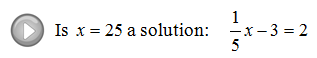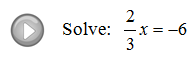Showing posts with label elementary algebra. Show all posts
Showing posts with label elementary algebra. Show all posts

## Wednesday, November 7, 2012

### Solving Linear Equations: Part II

In this section we will solve more complicated linear equations.  If you can, combine same side like terms first then opposite side like terms using the techniques that we have learned in part I. Caution: A common error is to add or subtract a term on the same side of the equal sign.  Only use the opposite operation on opposite sides of the equal sign.
Solve.
Not all equations work out to have a single solution.  Some have infinitely many solutions such as x = x.  Here any number the we chose for x will produce a true statement.  Also, some equations have no solution such as x + 1 = x.

Contradiction – An equation that will always be false has no solution.
Identity – An equation that will always be true has any real number, R, as a solution.
It is quite common to encounter linear equations that require us to distribute before combining like terms.

Solve.
Literal equations are difficult for many people because there will be more than one variable.  Just remember that the letters are place holders for some number, so the steps for solving are the same.  Isolate the variable that it asks us to solve for.

Word Problem: The internet connection at the hotel costs $5.00 to log on and$0.20 a minute to access.  If Joe's total bill came to $18.00 then how many minutes did Joe spend on the internet? (Set up an algebraic equation and solve it) Video Examples on YouTube: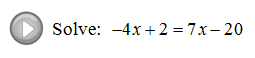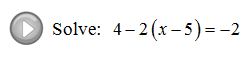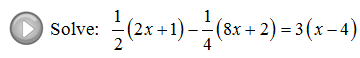J. Redden on G+ ## Saturday, November 3, 2012 ### Solving Linear Equations: Part I One method for solving a linear equation that looks like 2x + 3 = 13 is to simply guess and check. What value can I substitute in for x so that the result will be 13? After some thought it should be easy to see that x must equal 5 because 2 * 5 + 3 = 10 + 3 = 13. However, guessing at solutions to linear equations becomes very difficult when the numbers are not so nice. When solving, the idea is to use the properties of equality to isolate the variable. Tip: We can always check by substituting our solution into the original equation to see if we obtain a true statement. If you have time on an exam you should always check. Solve for x. Word Problem: Maria scored 7 more points than Jose on the first mathematics exam. If Maria's total score was 95 what was Jose's total. (Set up an algebraic equation and solve it) Tip: You can save a step if you multiply both sides of the equation by the reciprocal of the coefficient. When solving, apply the addition or subtraction property of equality first. Next apply the multiplication or division property of equality as needed to isolate the variable. Solving General Two Step Equations Steps for solving general linear equations of the form$ ax + b = c$. $$2x + 3 = 13$$ Add$-3$on both sides of the equation. $$2x = 10$$ Divide both sides by$2$. $$x = 5$$ Check:$2(5) + 3 = 10 + 3 = 13\$ ✔

Solve for x.